# HSSlive: Plus One & Plus Two Notes & Solutions for Kerala State Board

## BSEB Class 9 Maths Chapter 7 Triangles Ex 7.4 Textbook Solutions PDF: Download Bihar Board STD 9th Maths Chapter 7 Triangles Ex 7.4 Book AnswersBSEB Class 9 Maths Chapter 7 Triangles Ex 7.4 Textbook Solutions PDF: Download Bihar Board STD 9th Maths Chapter 7 Triangles Ex 7.4 Book Answers

BSEB Class 9th Maths Chapter 7 Triangles Ex 7.4 Textbooks Solutions and answers for students are now available in pdf format. Bihar Board Class 9th Maths Chapter 7 Triangles Ex 7.4 Book answers and solutions are one of the most important study materials for any student. The Bihar Board Class 9th Maths Chapter 7 Triangles Ex 7.4 books are published by the Bihar Board Publishers. These Bihar Board Class 9th Maths Chapter 7 Triangles Ex 7.4 textbooks are prepared by a group of expert faculty members. Students can download these BSEB STD 9th Maths Chapter 7 Triangles Ex 7.4 book solutions pdf online from this page.

## Bihar Board Class 9th Maths Chapter 7 Triangles Ex 7.4 Books Solutions

 Board BSEB Materials Textbook Solutions/Guide Format DOC/PDF Class 9th Subject Maths Chapter 7 Triangles Ex 7.4 Chapters All Provider Hsslive

## How to download Bihar Board Class 9th Maths Chapter 7 Triangles Ex 7.4 Textbook Solutions Answers PDF Online?

2. Click on the Bihar Board Class 9th Maths Chapter 7 Triangles Ex 7.4 Answers.
3. Look for your Bihar Board STD 9th Maths Chapter 7 Triangles Ex 7.4 Textbooks PDF.
4. Now download or read the Bihar Board Class 9th Maths Chapter 7 Triangles Ex 7.4 Textbook Solutions for PDF Free.

Find below the list of all BSEB Class 9th Maths Chapter 7 Triangles Ex 7.4 Textbook Solutions for PDF’s for you to download and prepare for the upcoming exams:

## BSEB Bihar Board Class 9th Maths Solutions Chapter 7 Triangles Ex 7.4

Question 1.
Show that in a right angled triangle, the hypotenuse is the longest side.
Solution:
Let ABC be a right angled A in which ∠ABC = 90°.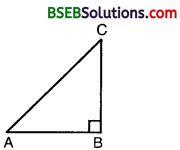But ∠ABC + ∠BCA + ∠CAB = 180°
⇒ 90° + ∠BCA + ∠CAB = 180°
⇒ ∠BCA + ∠CAB = 90°
⇒ ∠BCA and ∠CAB are acute angles
⇒ ∠BCA < 90° and ∠CAB < 90°
⇒ ∠BCA < ∠ABC and ∠CAB < ∠ABC ⇒ AC > AB and AC > BC
[∵ Side opp. to greater angle is larger]
⇒ In a right triangle, the hypotenuse is the longest side.

Question 2.
In figure, sides AB and AC of ∆ ABC are extended to points P and Q respectively. Also, ∠PBC < ∠QCB. Show that AC > AB.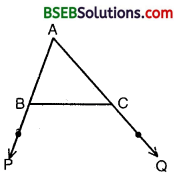Solution:
Since ∠PBC < ∠QCB ⇒ – ∠PBC > – ∠QCB
⇒ 180° – ∠PBC > 180° – ∠QCB
∠ABC > ∠ACB
⇒ AC > AB [∵ Side opp, to greater angle is larger]

Question 3.
In figure, ∠B < ∠A and ∠C < ∠D. Show that AD < BC.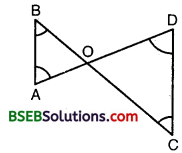Solution:
Since ∠B < ∠A and ∠C < ∠D
∴ AO < BO and OD < OC
[∵Side opp. to greater angle is larger]
AO + OD < BO + OC

Question 4.
AB and CD are respectively the smallest and longest sides of a quadrilateral ABCD (see figure). Show that ∠A > ∠C and ∠B > ∠D.Solution:
ABCD is a quadrilateral such that AB is its smallest side and CD is its largest side.
Join AC and BD.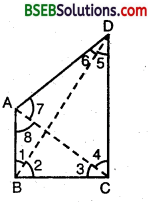Since AB is the smallest side of quadrilateral ABCD.
∴ In ∆ ABC, we have BC > AB ⇒ ∠8 > ∠3
[∵ Angle opp. to longer side is greater] Since CD is the longest side of quadrilateral ABCD.
∴ In A ACD, we have
⇒ ∠7 > ∠4 … (2)
[∵ Angle opp. to longer side is greater]
we get ∠8 + ∠7> ∠3 + ∠4
⇒ ∠A > ∠C
Again, in ∆ ABD. we have
AD > AB [∵AB is the shortest side]
⇒ ∠1 > ∠6 … (3)
In ∆ BCD, we have
CD > BC [∵ CD is the longest side]
⇒ ∠2 > ∠5 … (4)
Adding (3) and (4), we get
∠1 + ∠2 > ∠5 + ∠6 ⇒ ∠B > ∠D
Thus, ∠A > ∠C and ∠B > ∠D.

Question 5.
In figure, PR > PQ and PS bisects ∠QPR. Prove that ∠PSR > ∠PSQ.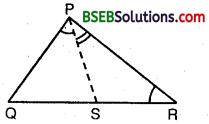Solution:
In ∆ PQR, we have
PR > PQ [Given]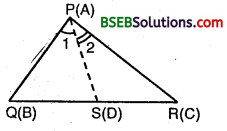⇒ ∠PQR > ∠PRQ [∵ Angle opp. to larger side is greater]
⇒ ∠PQR + ∠1 > ∠PRQ + ∠1 [Adding ∠1 on both sides]
⇒ ∠PQR + ∠1 > ∠PRQ + ∠2 [∵ PS is the bisector of ∠P ∴∠1 = ∠2 ]
Now, in ∆s PQS and PSR,
we have ∠PQR + ∠1 + ∠PSQ = 180°
and ∠PRQ + ∠2 + ∠PSR = 180°
⇒ ∠PQR + ∠1 = 180° – ∠PSQ
and ∠PRQ + ∠2 = 180° – ∠PSR
∴ 180° – ∠PSQ > 180° – ∠PSR [From (1)]
⇒ – ∠PSQ > – ∠PSR
⇒ ∠PSQ < ∠PSR i.e., ∠PSR > ∠PSQ.

Question 6.
Show that of all line segments drawn from a given point not on it, the perpendicular line segment is the shortest.
Solution:
Lot P be any point not on the straight line l. PM ⊥ l and N is any point on l other than M.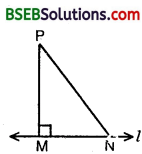In ∆ PMN, we have
∠M = 90°
⇒ ∠N < 90°

[ ∵ ∠M = 90° ⇒ ∠MPN + ∠PNM = 90°
⇒ ∠P + ∠N – 90° ⇒ ∠N < 90°]
⇒ ∠N < ∠M
⇒ PM < PN [∵ Side opp. to greater angle is larger]
Hence, PM is the shortest of all line segments from P to AB.

## Bihar Board Class 9th Maths Chapter 7 Triangles Ex 7.4 Textbooks for Exam Preparations

Bihar Board Class 9th Maths Chapter 7 Triangles Ex 7.4 Textbook Solutions can be of great help in your Bihar Board Class 9th Maths Chapter 7 Triangles Ex 7.4 exam preparation. The BSEB STD 9th Maths Chapter 7 Triangles Ex 7.4 Textbooks study material, used with the English medium textbooks, can help you complete the entire Class 9th Maths Chapter 7 Triangles Ex 7.4 Books State Board syllabus with maximum efficiency.

## FAQs Regarding Bihar Board Class 9th Maths Chapter 7 Triangles Ex 7.4 Textbook Solutions

#### Can we get a Bihar Board Book PDF for all Classes?

Yes you can get Bihar Board Text Book PDF for all classes using the links provided in the above article.

## Important Terms

Bihar Board Class 9th Maths Chapter 7 Triangles Ex 7.4, BSEB Class 9th Maths Chapter 7 Triangles Ex 7.4 Textbooks, Bihar Board Class 9th Maths Chapter 7 Triangles Ex 7.4, Bihar Board Class 9th Maths Chapter 7 Triangles Ex 7.4 Textbook solutions, BSEB Class 9th Maths Chapter 7 Triangles Ex 7.4 Textbooks Solutions, Bihar Board STD 9th Maths Chapter 7 Triangles Ex 7.4, BSEB STD 9th Maths Chapter 7 Triangles Ex 7.4 Textbooks, Bihar Board STD 9th Maths Chapter 7 Triangles Ex 7.4, Bihar Board STD 9th Maths Chapter 7 Triangles Ex 7.4 Textbook solutions, BSEB STD 9th Maths Chapter 7 Triangles Ex 7.4 Textbooks Solutions,
Share: